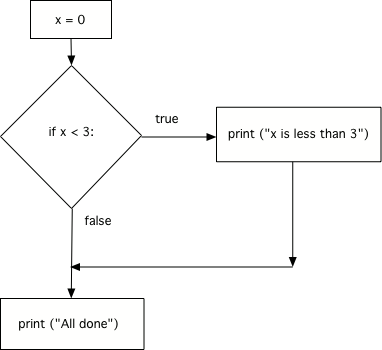The if Statement¶

In Python, we test data and execute instructions if the test is true using an if statement. An if statement includes a logical expression which is the ‘test.’ A logical expression is one that is either true or false. This is also called a Boolean expression. An example of a logical expression is x < 3. The if statement is followed by a colon if x < 3: and a block of code. The colon (:) at the end of the if statement is required. The block of code includes the instructions to execute if the test is true. The block of code includes all the statements that are indented following the if statement. If the test is true, execute the statement or statements in the block following the if will be executed. If the test isn’t true (is false) then execution will skip the block following the if and continue with the next statement following the block after the if statement.

Run the code below with x set to 0 and then change x to 4 and see how the output differs depending on the value of x.

The figure below is called a flowchart. It shows the execution paths for a program. The diamond shape contains the logical expression and shows the path that the execution takes if the logical expression (also called the condition) is true as well as the path if the logical expression is false. Notice that it will only execute the statements in the indented block if the logical expression was true. If the logical expression was false, execution will skip the code in the indented block and resume with the next statement.Figure 3: Flow of execution for an if statement